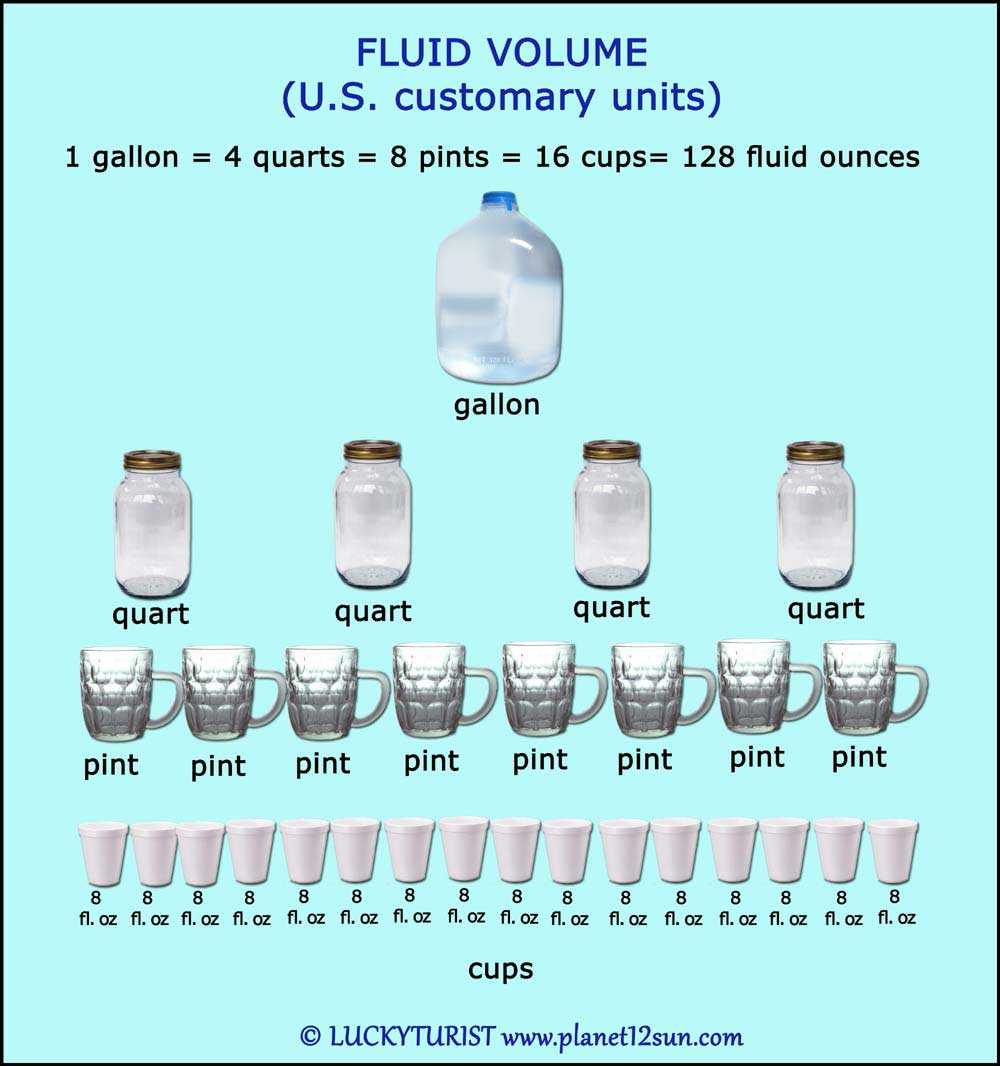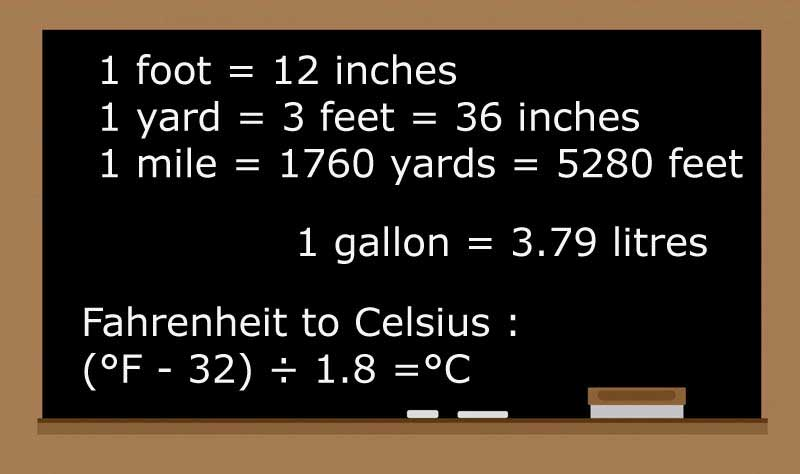## Volume

The volume of an object is a measure of the amount of space occupied by that object (not to be confused with mass).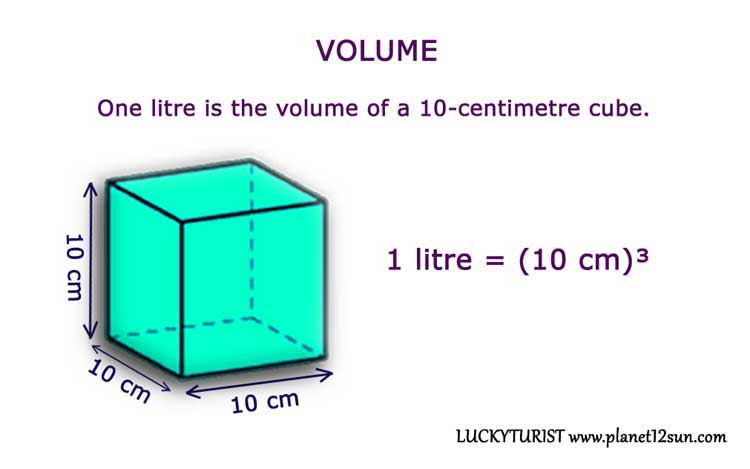### Metric System

In the International System of Units (SI), the standard unit of volume is the cubic metre (m³). The metric system also includes the litre (L) as a unit of volume, where one litre is the volume of a 10-centimetre cube.

1 litre = (10 cm)³ = 1000 cubic centimetres = 0.001 cubic metres
1 cubic metre = 1000 litres
1 hectoliter (hl) = 100 litres

Small amounts of liquid are often measured in millilitres.

1 millilitre = 0.001 litres = 1 cubic centimetre.

Examples:
Milk, soda and other drinks are often sold in litres.
20 drops of water droplets makes about 1 millilitre.
A Megaliter is a million litres (1,000,000 L). Useful for measuring large quantities of water, such as in lakes and dams.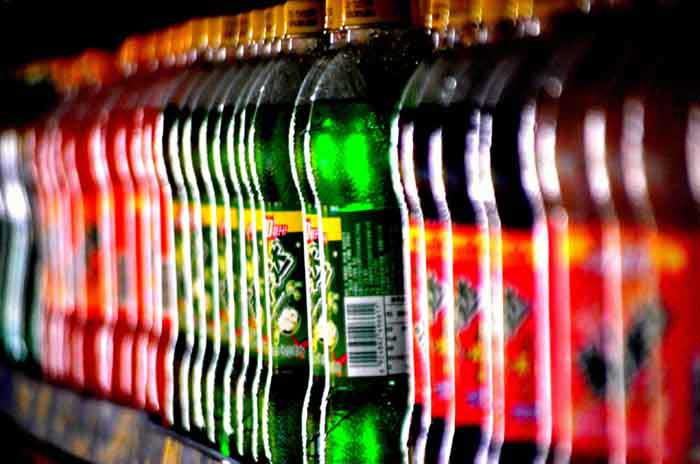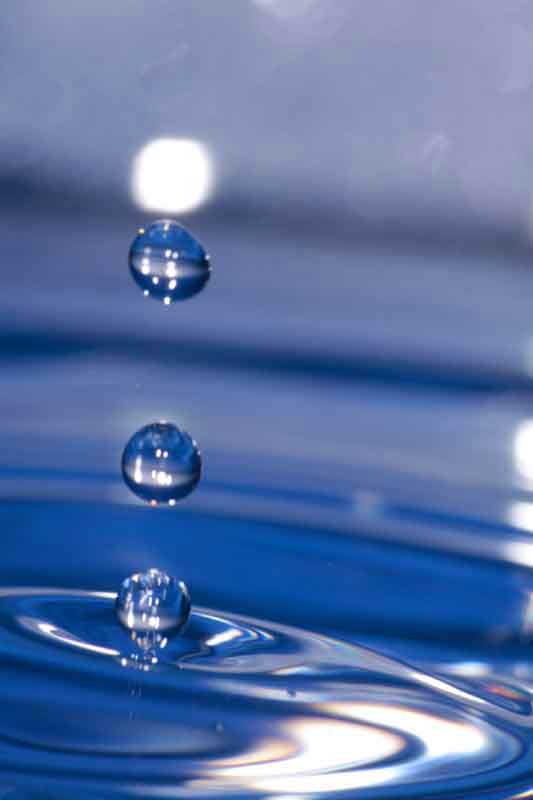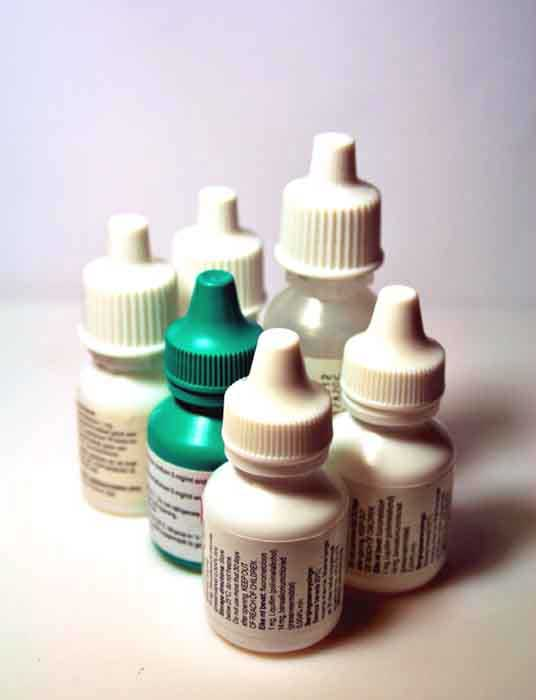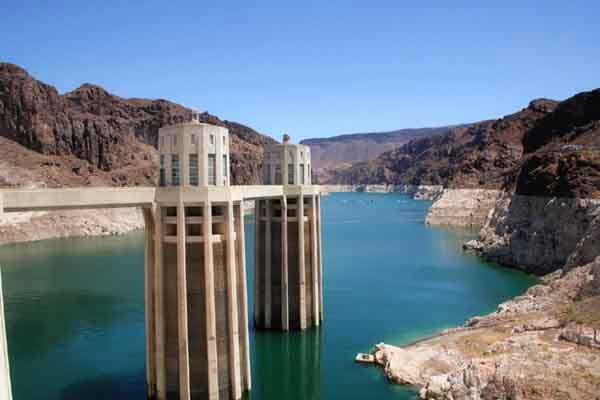Any unit of length gives a corresponding unit of volume, namely the volume of a cube whose side has the given length. For example, a cubic centimetre (cm³) would be the volume of a cube whose sides are one centimetre (1 cm) in length.

### US Standard Units (English Units, US Customary Units)

Traditional units are still in encountered in some countries: Imperial units such as the gallon or the fluid ounce were in widespread use within the British Empire. Some of them are still popular in the United States, which also uses units like the bushel, or the quart, the cup and the teaspoon (in cooking recipes, for example).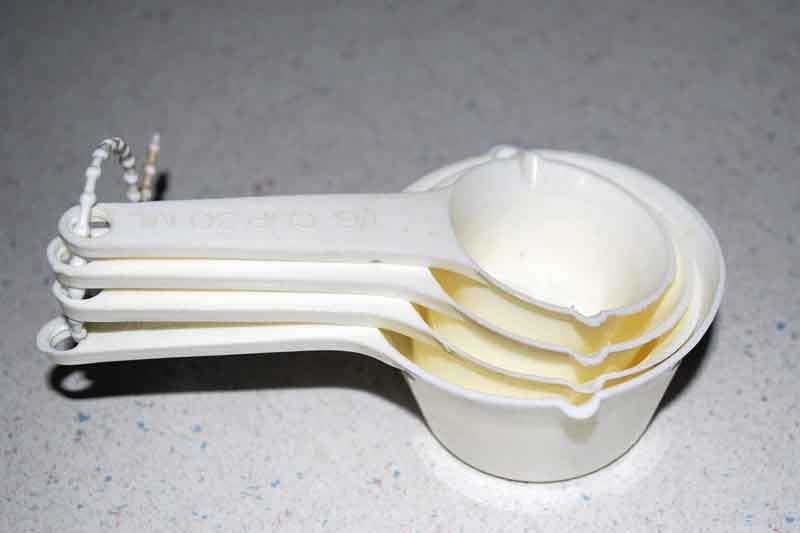Common volume units are the teaspoon, tablespoon (3 teaspoons), fluid ounce (two tablespoons), cup (8 ounces), pint (2 cups, or 16 fluid ounces), quart (2 pints, or 32 fluid ounces), US gallon (16 cups, 128 fluid ounces, or 3.8 liters).

A quart is a quarter of a gallon.
1 gallon = 4 quarts = 8 pints = 16 cups= 128 fluid ounces
1 US cup = 8 US fluid ounces
1 US pint = 2 US cups = 16 US fluid ounces
1 US quart = 2 US pints